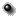OMNIWeb Plus Browser
to select different options to produce plots, listings or output files.
SW Wind hourly Magnetic Field, SWE Plasma definitive, cross_normalized (to SWE) plasma 3DP Data and their derived parameters

This new interface allows the OMNI users (https://omniweb.gsfc.nasa.gov/ow.html) to calculate more accurately a few "OMNI derived parameters" that are currently included in the OMNI2 dataset. These parameters are the Flow presure, Plasma Beta, Alfven Mach Number, Magnetosonic Mach Number, and Quasi-Invariant(QI). To create the new derived parameters we have taken into account the really measured values of the Wind/SWE Alpha Density.
Using this new interface, a user can compare these derived parameters: (1) as based on the Proton Density only, (2) as calculated by the technique used in the original OMNI database = Alpha Particles Density (Na) = 5% of Proton Density (Np); and (3) as calculated based on the Alpha Density real measurements (Na) along with the Proton Density (Np).
All details of the above parameters calculation can be found at the OMNI Web site https://omniweb.gsfc.nasa.gov/ftpbrowser/bow_derivation.html. We believe that the newly derived parameters (that are based on the Proton and real Alpha densities) can be more realistic for the periods when the SW plasma is significantly disturbed (e.g., during CME, magnetic storms, etc.) when the Alpha Density might be quite different from the values originally used in OMNI2 dataset (Na= 5% of Np).
Similar as in the OMNI2 database, we use here same assimptions for the ratios of: (1) Alpha (Ta) to Proton (Tp) temperatures as Ta/Tp = 4, (2) Electron Te = 1.4*10**5 and Alpha Paticles Speed (Va) to Proton (Vp) as Va = Vp.
Also, in addition to the Wind/SWE plasma parameters (that are used in OMNI), we now offer the Wind 3DP plasma parameters (that are cross-normalized to SWE definitive fit) and the derived parameters calculated similarly as we did for the Wind/SWE Plasma data. Note that the 3DP plasma data correlate pretty well with the SWE plasma data, but the 3DP data may have less data gaps for some intervals than the SWE plasma data.
In this new interface, we also added some advanced plotting options that provide much easier way to compare these derive parameters with each other for one type of parameter (up to four graphs on the one plot panel).
https://omniweb.gsfc.nasa.gov/ftpbrowser/ and
https://cdaweb.sci.gsfc.nasa.gov/ under spacecraft "Wind".

```Time span of data sets
SWE definitive  1995-01-01 - 2023-10-08
Plasma 3DP      1995-01-01 - 2023-10-09
```
Plot data List data Create file (file?)Select resolutionEnter start and stop times: (use format YYYYMMDD )
Start Stop

Magnetic field
 IMF Magnitude Avg, nT

SWE_definitive Plasma (Original parameters)
 Flow Speed, km/s Proton Density, n/cc Proton Temperature, K
 Na/Np Ratio Alpha Temperature, K
SWE Derived parameters based on Proton density
 Flow Pressure, nPa Plasma Beta Alven mach number
 Magnetosonic Mach num. Quasi-Invariant(QI)
SWE Plasma Derived parameters,( (based on Alpha Density = 5% Proton Density (used in OMNI)
 *Flow Pressure, nPa Plasma Beta Alven mach number
 Magnetosonic Mach num. Quasi-Invariant(QI)
SWE Plasma Derived parameters based on Protons and Real Alpha Density
 Flow Pressure Plasma Beta Alven mach number
 Magnsonic Mach num. Quasi-Invariant(QI)

Normalized (to SWE plasma) 3DP Plasma parameters
 Flow Speed, km/s Proton Density, n/cc Proton Temperature, K
 Na/Np Ratio Alpha Temperature, K
3DP derived parameters based on Proton Density
 Flow Pressure, nPa Plasma Beta Alven mach number
 Magnetosonic Mach num. Quasi-Invariant(QI)
3DP derived parameters,( based on Alpha Density = 5% Proton Density
 Flow Pressure,nPa Plasma Beta Alven mach number
 Magnsonic Mach num. Quasi-Invariant(QI)
3DP Derived parameters based on Protons and Alpha Density
 Flow Pressure, nPa Plasma Beta Alven mach number
 Magnsonic Mach num. Quasi-Invariant(QI)

* In the OMNI Data Base the Flow Pressure P (nPa) : = (1.67/10**6) * Np*V**2 * (1+ 4*Na/Np) for hours with non-fill Na/Np ratios and P (nPa) = (2.0/10**6) * Np*V**2 for hours with fill values for Na/Np.Advanced plot selections (optional)To show a few variables on one panel, please specify the number of variables( 1-4) in the table up[one type of variable, e.g Speed]:
and the same number in this select box:

 Y-axis Scale: Linear Log10 Character size(0.5-2.0): Plot Symbol: None Plus Asterisk Period Diamond Triangle Square X Symbol Size(0.1-4.0): Image size (pixels) X: Y:

 If you have any questions/comments about the OMNWeb Plus, contact Dr. Natalia Papitashvili,Mail Code 672, NASA/Goddard Space Flight Center, Greenbelt, MD 20771

NASA Official: Robert Candey (Robert.M.Candey@nasa.gov), Head of the Space Physics Data Facility